# JEE Main Kinematics 2D Previous Year Questions with Solutions

Kinematics is a branch of physics that deals with the geometric motion of the object without considering the force that causes the motion. Kinematics 2D is considering the motion of an object in 2 dimensions. The Kinematic equations are used to determine the unknown value of the object in motion when certain values are known. The same equation in Kinematics 2D is given in both x-direction and y-direction. The motion in x-direction and y-direction are considered independent of each other, except that they are related by the time.

## JEE Main Previous Year Solved Questions on Kinematics 2D

Q1: A river is flowing from west to east at a speed of 5 metres per minute. A man on the south bank of the river, capable of swimming at 10 metres per minute in still water, wants to swim across the river in the shortest time. He should swim in a direction.

1. due north
2. 30° east of north
3. 30° west of north
4. 60° east of north

Solution: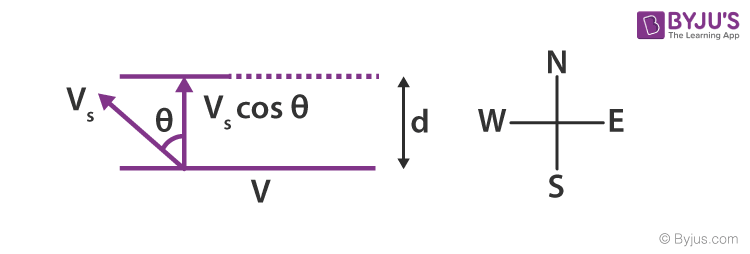Let the time taken to cross the river be t

t = d/Vs Cosθ

For the time t to be minimum, cosθ should be maximum

θ should be 0

Therefore swimmer should swim due north

Q2: A particle P is sliding down a frictionless hemispherical bowl. It passes the point A at t = 0. At this instant of time, the horizontal component of its velocity is v. A bead Q of the same mass as P is ejected from A at t = 0 along the horizontal string AB, with speed v. Friction between the bead and the string, may be neglected. Let tp and tq be the respective times taken by P and Q to reach point B.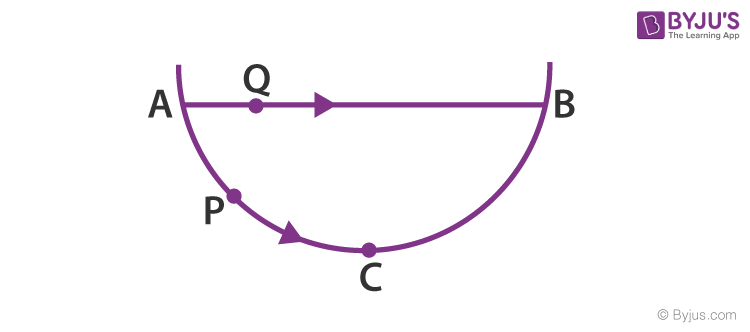1. tp ＜ tQ
2. tp ＞ tQ
3. tp = tQ
4. tp/ tQ = (length of arc ABC/ length of chord AB)

Solution

Horizontal displacements of the particle (P) and bead (Q) are equal. Bead Q moves along AB with a constant velocity v. For particle P, motion between AC will be under acceleration while between CB will be under retardation. The horizontal component of its velocity may be greater than or equal to v. It will not be less than v. Obviously, tP < tQ.

Q3: A train is moving along a straight line with a constant acceleration ‘a’. A girl standing in the train throws a ball forward with a speed of 10 m/s, at an angle of 600 to the horizontal. The girl has to move forward by 1.15 m inside the train to catch the ball back at the initial height. The acceleration of the train, in m/s2, is

Solution :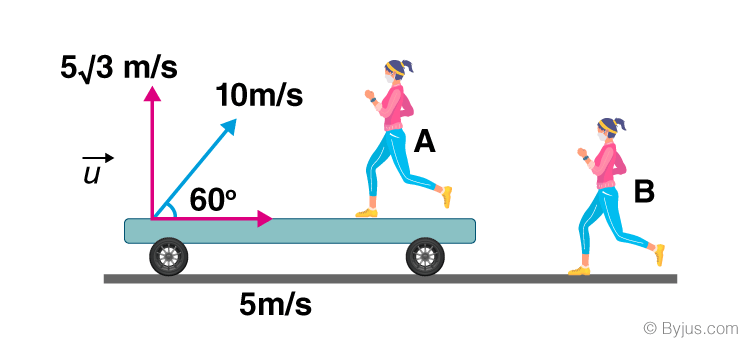R = ½ at2 + 1.15

u2sin 2θ/g = ½ a (2usin θ/g)2

$\frac{100 \times \sqrt{3}/2}{10} = \frac{1}{2}a\left (\frac{4\times 100\times 3/4}{100} \right )+1.15$ $5\sqrt{3} = \frac{3a}{2} + 1.15$ $2\times 5\sqrt{3}=3a+2.30$ $10\sqrt{3}=3a+2.30$ $10\sqrt{3}-2.30=3a$

10 x 1.73 – 2.3 = 3a

17.3 – 2.3 = 3a

15/3 = a

a = 5m/s2

Q4: A rocket is moving in a gravity-free space with a constant acceleration of 2 ms-2 along positive x-direction (see figure). The length of a chamber inside the rocket is 4m. A ball is thrown from the left end of the chamber in positive x-direction with a speed of 0.3 m/s relative to the rocket. At the same time, another ball is thrown in negative x-direction with a speed of 0.2 ms-1 from its right end relative to the rocket. The time in seconds when the two balls hit each other is

Solution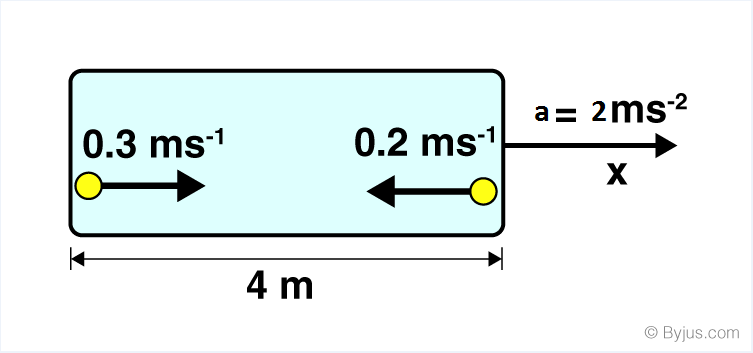The rocket moves in the direction of the left ball.

For the ball thrown from the left end

u1=0.3 m/s

Therefore the relative acceleration a1 = -2m/s2

Let us consider S1=x and t1= t

Since, S1 = u1t1 + ½ a1t12

Therefore x = 0.3t – (½)x 2t2

x = 0.3t – t2 ——–(1)

For the ball thrown from the right end

u2=0.2 m/s

Therefore the relative acceleration a2 = 2m/s2

Let us consider S2=4-x and t2= t

Since, S2 = u2t2 + ½ a2t22

Therefore 4-x = 0.2t – ½ 2t2 ——–(2)

Substituting the value of x from equa (1) in equa (2) we get

4 – (0.3t – t2) = 0.2t +t2

4 – 0.3t+t2 = 0.2t+t2

4 = 0.5t

t = 4/0.5 = 8 sec

Q5: A boat which has a speed of 5 km/hr in still water crosses a river of width 1km along the shortest possible path in 15 minutes. The velocity of the river in the shortest time. The velocity of river water in km/hr is

1. 1
2. 3
3. 4
4. $\sqrt{41}$

Solution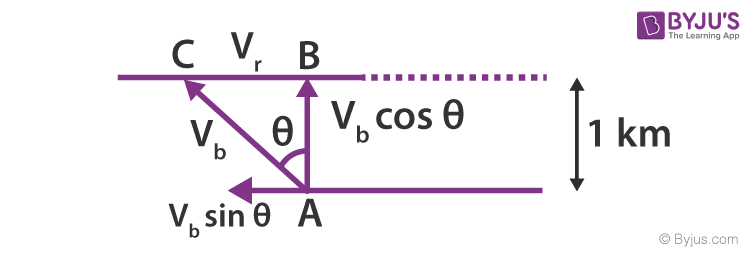Time t = 15 min = 15/60 = ¼ hour

Velocity = distance /time

Vbcosθ= 1/(¼) =4km/h

5cosθ=4km/h

cosθ= ⅘

Sinθ = ⅗

Consider the triangle of velocity ABC,

Vr/Vb = ⅗

Vr/5 = ⅗

Or Vr = 3km/h

Q6: In 1.0 sec a particle goes from point A to point B, moving in a semicircle of radius 1.0 m as shown in the figure. The magnitude of the average velocity is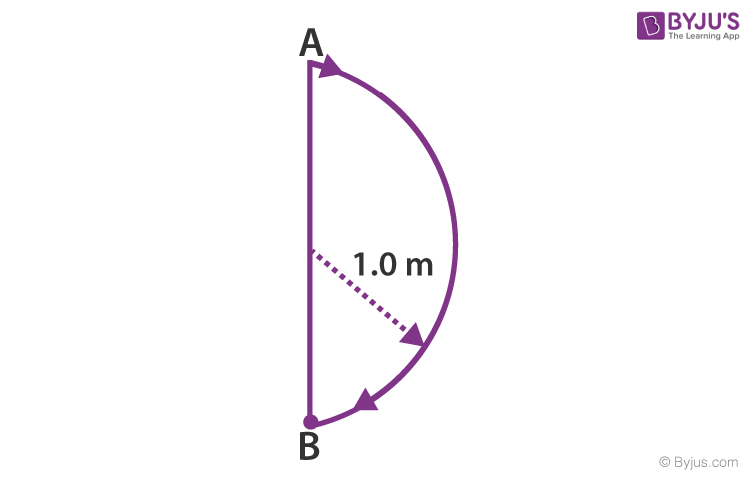1. 3.14 m/sec
2. 2.0 m/sec
3. 1.0 m/sec
4. zero

Solution:

Average velocity = displacement/ time

Average velocity = 2r/t = 2 x 1/1 = 2m/s

Q7: Aeroplanes A and B are flying with constant velocity in the same vertical plane at angles 300 and 600 with respect to the horizontal, respectively as shown in the figure. The speed of A is $100\sqrt{3}$ m/s. At time t = 0 s, an observer in A finds B at a distance of 500 m. This observer sees B moving with a constant velocity perpendicular to the line of motion of A. If at t = t0, A just escapes being hit by B, t0in seconds is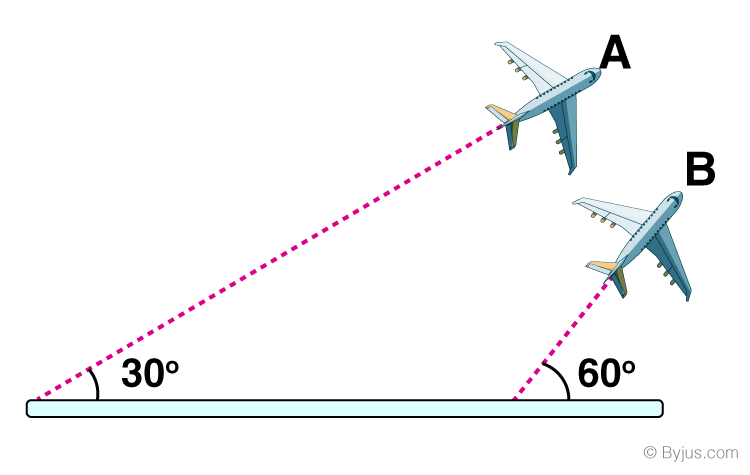Solution

As observed from A, B moves perpendicular to the line of motion of A. It means the velocity of B along A is equal to the velocity of A.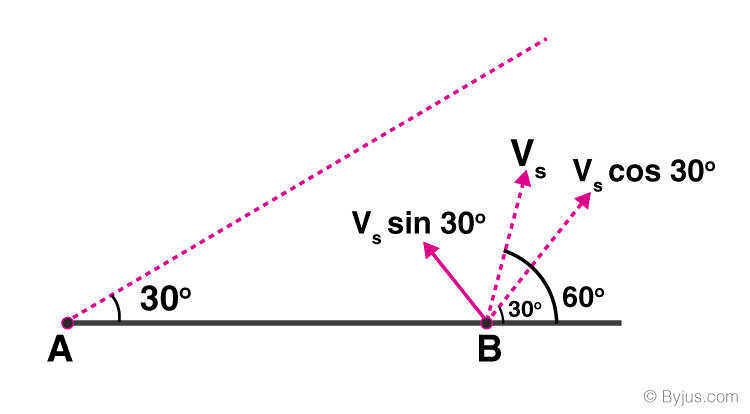VA = $100\sqrt{3}$ =VB Cos 300

Therefore,VB = 200m/s

t0 = 500/ VB Cos 300

= 500/ 200x (½)

= 5 sec

Q8: A ball is projected from the ground at an angle of 450 with the horizontal surface. It reaches a maximum height of 120 m and returns to the ground. Upon hitting the ground for the first time, it loses half of its kinetic energy. Immediately after the bounce, the velocity of the ball makes an angle of 300 with the horizontal surface. The maximum height it reaches after the bounce, in metres, is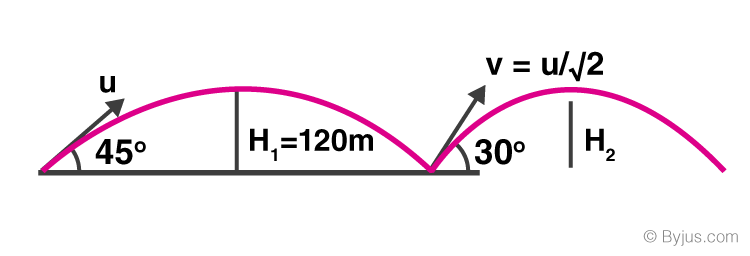Solution

H1 = u2sin245/2g = 120

u2/2g = 120—————— (1)

When half of the kinetic energy is lost $v=u/\sqrt{2}$

H2 = ($u/\sqrt{2}$)2sin230/2g = u2/16g——-(2)

From (1) and (2)

H2 = H1/4 = 30 m

Q9: A sphere is rolling without slipping on a fixed horizon­tal plane surface. In the figure, A is the point of contact, B is the centre of the sphere and C is a topmost point, Then,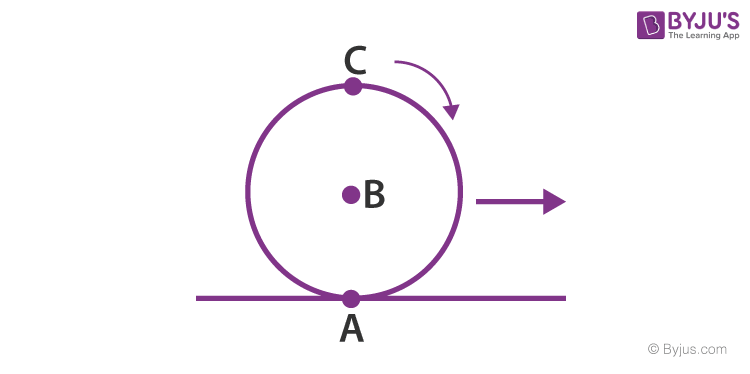1. $\vec{V_{c}}-\vec{V_{A}}=2(\vec{V_{B}}-\vec{V_{c}})$
2. $\vec{V_{c}}-\vec{V_{A}}=\vec{V_{B}}-\vec{V_{c}}$
3. $\left | \vec{V_{c}}-\vec{V_{A}} \right |=2\left | \vec{V_{B}}-\vec{V_{c}} \right |$
4. $\left | \vec{V_{c}}-\vec{V_{A}} \right |=2\left | \vec{V_{B}}-\vec{V_{c}} \right |$

Solution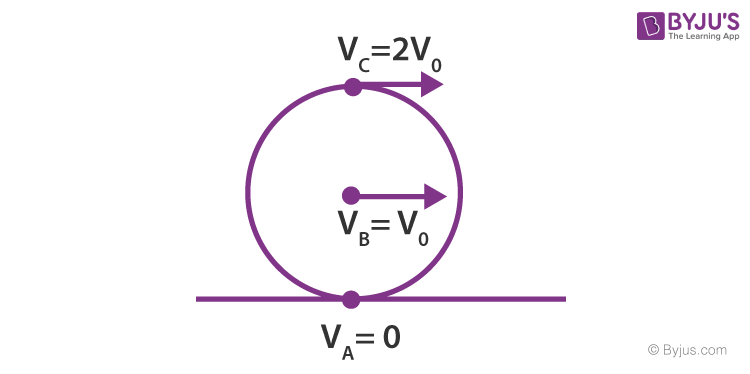By the concept of rolling

Velocity at point A is zero

If V0 is the velocity of centre of the sphere, then

$\vec{V_{c}}=2\vec{V_{0}}$ $\vec{V_{B}}=\vec{V_{0}}$

VA= 0

$\vec{V_{c}}-\vec{V_{B}}=2\vec{V_{0}} -\vec{V_{0}} = \vec{V_{0}}$

And $\vec{V_{B}}-\vec{V_{A}}=\vec{V_{0}} -0 = \vec{V_{0}}$ $\vec{V_{C}}-\vec{V_{B}}=\vec{V_{B}}-\vec{V_{A}}$

Now $\left | \vec{V_{C}}-\vec{V_{A}} \right |=\left | 2\vec{V_{0}}-0 \right |=\left | 2\vec{V_{0}}\right |=2\left | \vec{V_{0}}\right |$

And $\left | \vec{V_{C}}-\vec{V_{A}} \right |=2\left | \vec{V_{B}}-\vec{V_{A}} \right |$

Q10: Two balls of different masses are thrown vertically upwards with the same speed. They pass through the point of projection in their downward motion with the same speed (Neglect air resistance). Is the statement true or false?

Solution:

v2= u2 – 2gH

As the body falls back to the point of projection, H=0

v2= u2 – 0 = u2

Or v = u

Hence the statement is true

Q11: A projectile fired from the ground follows a parabolic path. The speed of the projectile is minimum at the top of its path. Is the statement true?

Solution:

The horizontal velocity of projectile = ucosθ

It remains constant throughout the motion

The vertical velocity becomes zero at the top of the path

Therefore the velocity is minimum at the top

Hence the statement is true

Q12.Two identical trains are moving on rails along the equator on the earth in opposite directions with the same speed. They will exert the same pressure on the rails. Is the statement true?

Solution:

One train is moving in the direction of the earth’s rotation and the other moves in the opposite direction. Hence they will exert different pressures on the rails. The statement is false.

Q13: A particle moves in a circle of radius R. In half the period of revolution its displacement is………. and distance covered is ………

Solution: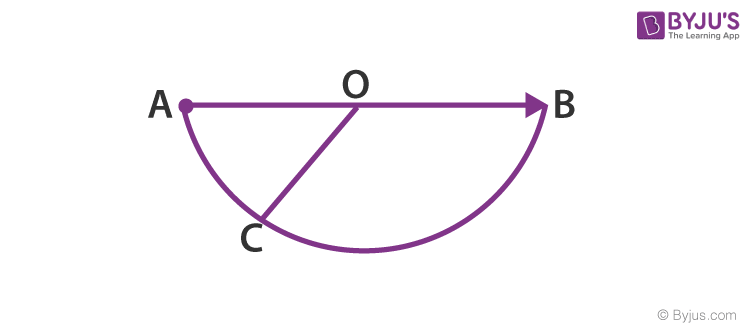Displacement AB = 2r

Distance ACB = 2πr/2 = πr

Q14: Four persons K, L, M, N are initially at the four corners of a square of side d. Each person now moves with a uniform speed V in such a way that K always moves directly towards L, L directly towards M, M directly towards N, and N directly towards K. The four persons will meet at a time …………..

Solution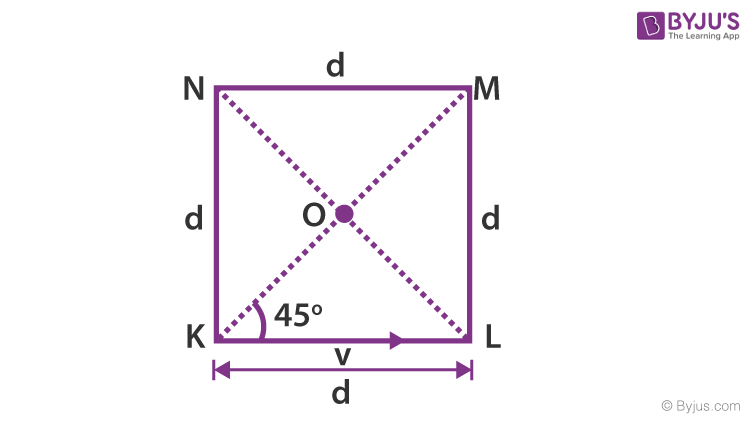It is inferred on the basis of symmetry that all four persons will meet at O, the centre of the square.

At any instant, the component velocity along

KO = vcos 450 = v/√2

Distance KO = dCos 450 = d/√2

Time taken = Distance KO/velocity along KO

Time Taken = (d/√2)/(v/√2)= d/v

Q15: The trajectory of a projectile in a vertical plane is y = ax – bx2, where a, b are constants, and x and y are respectively the horizontal and vertical distances of the projectile from the point of projection. The maximum height attained is……….and the angle of projection from the horizontal is

Solution:

y = ax – bx2

Comparing the given equation with

$y = (tan\theta )x -(\frac{g}{2u^{2}cos^{2}\theta })x^{2}$

Then a = tan Ө and b = $\frac{g}{2u^{2}cos^{2}\theta }$

Maximum height= a2/4b and angle of projection,

tan Ө =a

Ө = tan-1a